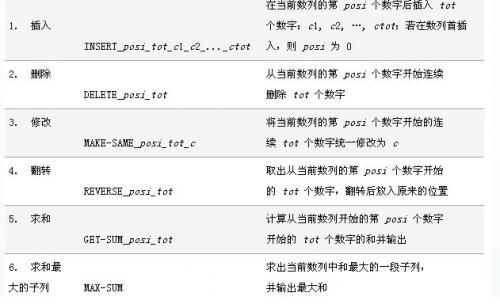# 题目大意

1. 向以$v$为根的子树的所有节点各插入$k$个数$z$（相当于每个节点存了一些数）
2. 询问某个节点$v$中有多少个数$x\ xor\ y\le z$，其中$y,z$是给出的
3. 把整棵树的根换成某一个节点$v$

$n,m\le 140000$

# 题目大意# 题目大意

• $1\ a\ b\ c$ 在第$a$个位置到第$b$个位置，每个位置加入一个数$c$。
• $2\ a\ b\ c$ 询问从第$a$个位置到第$b$个位置，第$c$大的数是多少。
###### Your browser is out-of-date!

Update your browser to view this website correctly. Update my browser now

×